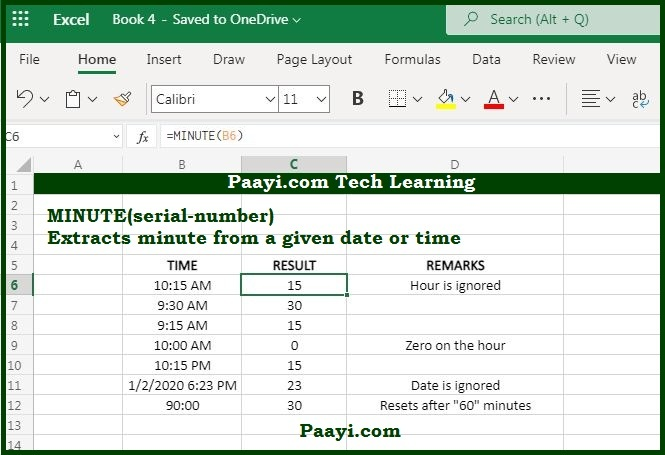# Learn How to Use Microsoft Excel MINUTE Function

Written by | 0 Comments | 576 Views

In this article, you will learn how to use the Microsoft Excel MINUTE function and its prime function in Microsoft Excel. You will also get to know the Microsoft Excel MINUTE function return value and syntax with the help of some examples.

## Microsoft Excel MINUTE Function

The MS Excel MINUTE function is used to extract minutes as a number from time. That means the MINUTE function returns the minute as a number from a time from 0 - 59. Let's say, for example, with the given time 10:45 AM, and MINUTE will return 45. So with the MINUTE function, you can extract the minute into a cell or can also feed the result into another function like the TIME function.

### MINUTE Function Return Value

It will return the number between 0 to 59.

### MINUTE Function Syntax

= MINUTE(serial-number)

Where:

• serial-number: A valid Excel date or time.

## How to Use Microsoft Excel MINUTE Function?As we know, the MINUTE function extracts the minute from a time as a number from 0 to 59; let's understand this as an example given below:

Example 1:

• = MINUTE("10:45 AM") - This will return the value 45.
• = MINUTE("09:15 PM") - This will return the value 15.

Example 2: You can also use the MINUTE function to extract the minute into a cell or feed the result into another function like the TIME function. Let's say the time in cell A1 is 6:45 PM, and you can create a new time including 45 minutes as given below:

• =TIME(8, MINUTE(A1), 0) - This will return the time 8:45 PM.